>>

Login or Register   Potential and kinetic energy
 New User Register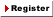Login Member: Email: Password:
 Site Search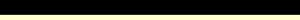Advance Search

Other animationsUnderstand pressure through cooker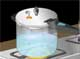Animation product List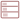Row formatGrid format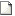List format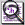Overview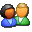(For age - group : Above 16 )

This science animation Introduces you basic forms of energy - potential and kinetic energy, it establishes relationship between them, then analyses gravitation, spring, molecular formation in terms of these energies. The detailed presentation clearly brings through the concept of PE & KE.Product - Animation
 Size/Duration Subscribe Preview / Trailer Size (KB) 1012 US\$ 16.00    2.00 Rs.158.00  Check price in your currency Subscription Days = 30 Watch a preview (opens in separate window) Duration (hr:min:sec) 1:40:0 Add To Cart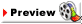Category : Science Type : Animation Animation Type : Advanced Total animation length: 1 hour 40 minutes Introduction to energy and it's types Relationship between potential and kinetic energy Examples of potential energy Relationship between force and potential energy Potential energy at atomic level Formation of hydrogen molecule How to calculate potential energy? What is kinetic energy? How to calculate kinetic energy? Various forms of energy Inter-conversion of energies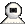SnapshotsUnderstanding potential energy through the animated examples of gravitational force and elasticity.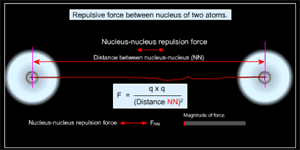At atomic level, the potential energy plays a significant part in the formation of molecules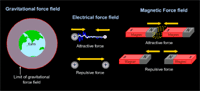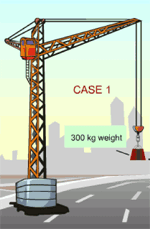All energies are derived from potential and kinetic energies.We come across energies in many formats - like thermal, light, chemical in our day-to-day life. Relationship between kinetic and potential energy through animated crane example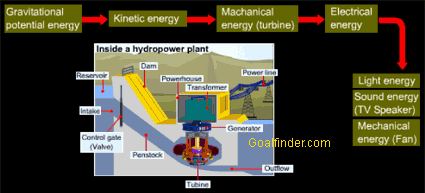Inter-conversion of energy using examples animated hydroelectric power plant and a pendulumDetails of the animation/ movie /software

This science animation follows a macro to micro path by probing deeper into various energies to unravel their common base - Potential and kinetic energy.

1. Introduction to energy and it's types: animation shows energy is everywhere around us in various forms.
2. Elastic, chemical, gravitational, mechanical are all forms of potential and kinetic energy
3. Establishes relationship between potential and kinetic energy through crane example
4. What is potential energy? Explained on basis of work
5. Examples of potential energy: F = -du/dx or force field always tries to lower PE.
• Case 1: Gravitational force - relationship between PE and position shown by ball and earth's surface example
• Case 2: Elastic force - stretching of a spring
6. Relationship between force and potential energy
7. Potential energy at atomic level
• Case 1 - Opposite charges
• Case 2 - Similar charges
8. Formation of hydrogen molecule - electron cloud - direction of rotation of electron.
9. Variation of potential energy with inter nuclear distance - bond formation - Potential well
10. How to calculate potential energy?
11. What is kinetic energy?
12. How to calculate kinetic energy?
13. Various forms of energy
14. Inter-conversion of energies: Explained in detail by taking each position of pendulum separately and analysing the forces acting on it at that position.
15. Application of conversion of energy: Hydroelectric Power Plant from potential-kinetic-mechanical-electric-sound or light or mechanical energy.
16. Explanatory notes Section :
• Potential energy function or how to calculate PE if force is known?
• Why Negative sign is there in formula of PE ?Related Products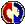Buyers Feedback:Give your comments